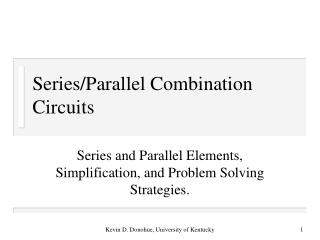# Series/Parallel Combination Circuits - PowerPoint PPT PresentationDownload PresentationSeries/Parallel Combination Circuits

Series/Parallel Combination Circuits
Download Presentation## Series/Parallel Combination Circuits

- - - - - - - - - - - - - - - - - - - - - - - - - - - E N D - - - - - - - - - - - - - - - - - - - - - - - - - - -
##### Presentation Transcript

1. Series/Parallel Combination Circuits Series and Parallel Elements, Simplification, and Problem Solving Strategies. Kevin D. Donohue, University of Kentucky

2. Series and Parallel Elements • Two elements are connected in series if and only if they have a common node to which no other elements are attached. • Two elements are connected in parallel if and only if they have common nodes at both terminals. R1 R2 Is R3 R2 R3 Vs R1 Kevin D. Donohue, University of Kentucky

3. Equivalent Resistance • Series and parallel resistors can be combined into one element with an equivalent resistance. • Equivalent resistance refers to a single resistance replacing a multiple resistor subcircuit such that it draws the same current and produces the same voltage drop. • Apply Ohm’s law to derived formula for finding equivalent resistance. Kevin D. Donohue, University of Kentucky

4. R1 R2 Req  RN  Req RN R1 R2 Series and Parallel Equivalents Kevin D. Donohue, University of Kentucky

5. Series and Parallel Sources • Voltage sources in series can be combined into a single voltage source: Va Vb Va+Vb+Vc Vc • Current sources in parallel can be combined into a single current source: Ia Ib Ic Ia + Ib + Ic Kevin D. Donohue, University of Kentucky

6. Examples Simplify complex circuits containing independent sources and resistors to a minimum number of equivalent elements. Kevin D. Donohue, University of Kentucky

7. Examples • Find voltages and currents in all branches of circuits containing resistors and independent sources through simplifying and expanding the circuit • Voltages and currents in the original circuit can be determined by • Finding currents and voltages of the simplified circuit • Expanding back to the original configuration while applying current and voltage division to determine their current and voltage values. Kevin D. Donohue, University of Kentucky

8. Single Loop Circuits with Dependent Sources Since current is the the same throughout the entire loop, express all unknowns in terms of the loop current. Find Vo in circuit below: 120  100Vd 2  + Vd - + Vo - 3  6 V Kevin D. Donohue, University of Kentucky

9. Single Node-Pair Circuits with Dependent Sources Since voltage is the the same across every element, express all unknowns in terms of the node voltage difference. Find Io in circuit below: Io Id 0.8Id 2  6 A 3  Kevin D. Donohue, University of Kentucky